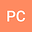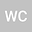Higher Dimensional Hermite-Hadamard Inequality for Semiconvex Functions of Rate $(k_1,k_2,…,k_n)$ on the Co-ordinates and Optimal Mass Transportation
••• Ping Chen,
• Wing-Sum Cheung
Ping Chen
Jiangsu Second Normal University

Corresponding Author:[email protected]

Author ProfileWing-Sum Cheung
University of Hong Kong Department of Mathematics
Author Profile## Abstract

In this paper, we give a new higher dimensional Hermite-Hadamard inequality for a function $f:\prod\limits_{i=1}^n[a_i,b_i]\subset\mathbb{R}^n\rightarrow\mathbb{R}$ which is semiconvex of rate $(k_1,k_2,…,k_n)$ on the co-ordinates. This generalizes some existing results on Hermite-Hadamard inequalities of S.S. Dragomir. In addition, we explain the Hermite-Hadamard inequality from the point of view of optimal mass transportation with cost function $c(x,y):=f(y-x)+\sum_{i=1}^n\frac{k_i}{2}\big|x_i-y_i\big|^2$, where $f(\cdot):\prod\limits_{i=1}^n[a_i,b_i]\rightarrow[0,\infty)$ is semiconvex of rate $(k_1,k_2,…,k_n)$ on the co-ordinates and $x=(x_1,x_2,…,x_n)$, $y=(y_1,y_2,…,y_n)\in\prod\limits_{i=1}^n[a_i,b_i]$. Furthermore, by using the higher dimensional Hermite-Hadamard inequality, we compare the transport cost in different transport models on the sphere $\mathbb{S}^2$.
14 Aug 2020Submitted to Mathematical Methods in the Applied Sciences
17 Aug 2020Submission Checks Completed
17 Aug 2020Assigned to Editor
24 Aug 2020Reviewer(s) Assigned
02 Jan 2021Review(s) Completed, Editorial Evaluation Pending
04 Jan 2021Editorial Decision: Revise Major